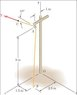physicsss
Before a telephone cable is strung, rope BAC is tied to the stake at B and is passed over a pulley at A. Knowing that portion AC of the rope lies in a place parallel to the xy plane and that the tension T in the rope is 124 N, determine the moment about O of the resultant force exerted on the pulley by the rope.

How do I find the resultant force?

#### Attachments

•Picture1.jpg
12.4 KB · Views: 632

Homework Helper
Draw a free-body diagram.
Consider the plane BAC, which is parallel to the x-y plane.
You have tensions in the ropes AB and AC. You know, or can work out, the angle between the two tensions. You should now be able to work out the resultant of the two tensions.

physicsss
I don't think plane BAC is parallel to the x-y plane. Only line AC is.

Homework Helper
You're right. I should wear my specs more often!

Mmmm ...

physicsss
So F from A to B is 124(1.5m i - 9m j + 1.8m k)/sqrt(1.5m^2+9m^2+1.8m^2)
and from F from A to C is 124N cos(10) i +124N*sin(10) j ?

Last edited:
Homework Helper
I'm doing it by vectors, atm, and I get the resultant force = 176N. (not checked)

I've got the resultant as a vector. But I cant' remember the formula for distance to a line from a point ( e.g. the origin). Any ideas ??

physicsss
My resultant force was 174.5 N, close enough?

Homework Helper
yeah, I approximated a bit, just to work through :)

physicsss
I got 137 i - 142 j + 1393 k for the moment...

Homework Helper

I think I'm doing something wrong with this formula I have for the distance from a point to a line :(

physicsss
Oops...it's 118 i - 142 j + 1279 k...my mistake...

Homework Helper
Well, then that could be about right. I got 1250 using the value of 176.17 for the resultant force.

I've still got a niggling point about the formala I used for the distance though.
Have you ever seen http://ericvesey.com/geometry/vectors.pdf#nameddest=10 [Broken] - bottom of page 5.

I tried to use it to compute the distance of the point B, in your sketch, from the origin and got zero!

Last edited by a moderator:
physicsss
Hmm forget what I said above...I didn't do it that way, I crossed the distance from the origin to the pulley with the resultance force in its i, j, k components:

(9 j + 1 k) X (142 i - 98.468 j + 24 k), and the resultant is 1325...